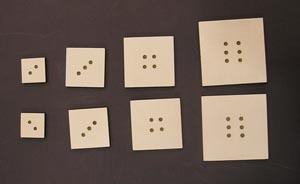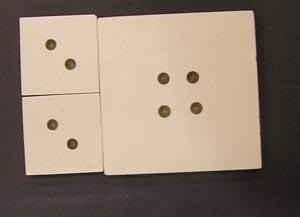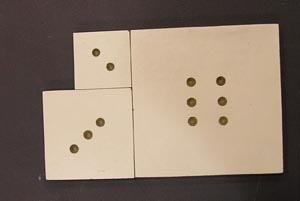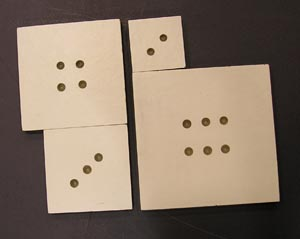Multiplication Tiles

It all adds up to multiplication.

Introduction

A set of square tiles can be made so that when two tiles are placed side by side they have the same width as a tile which is the product of the two numbers.A set of multiplication tiles.

Material

Wood a few mm thick which you can cut into squares.

A piece of log-log graph paper (Or the table below)

Assembly

Mark squares on the wood with a width that is proportional to the base ten logarithm of the number on the tile. Cut two tiles each of tiles 2,3,4,5,6,8,9,and 10.

Mark numbers on each tile.

To Do and Notice

Place two tiles side-by-side and find another single tile with the same width.Two 2 tiles are the same width as one 4 tile.A 3 tile and a 2 tile are the same width as a 6 tile.

You can also place two tiles together and find two other tiles width the same width.A 3 tile and a 4 tile are the same width as a 2 tile and a 6 tile.

What's Going On?

The tiles are cut so that the length of the side is the logarithm of the number on the tile.

If you add logarithms, log 2 + log 2 = log 4 the sum of the logarithms is the log of the product of the numbers.

We add logarithms by adding lengths, that is by placing tiles side-by-side.

A Scottsman named Napier, the "Fear of Murchison," invented the idea of logarithms. The sum of the logarithms of two numbers is the logarithm of the product of the numbers.

Log A + Log B = Log AB.

This great invention turned multiplication into addition. It increased the speed of mathematical computation as much as the invention of computers did in the 20'th century.

Math Root

Choose a size of the side for the number 10 block, perhaps 10 cm. Call this distance 1.

This table shows the relative sizes of the sides of the other numbered blocks.

 number size 10 1.0 9 0.95 8 0.90 6 0.78 5 0.70 4 0.60 3 0.48 2 0.30

As an alternate method of creating the squares you can use log-log graph paper.

Cut out squares with sides corresponding to each number.

 Scientific Explorations with Paul Doherty © 2004 22 October 2004